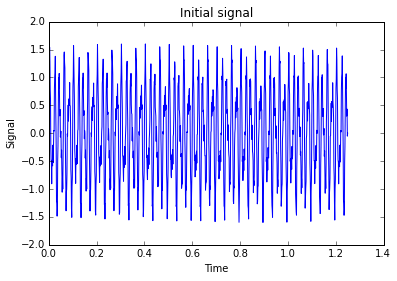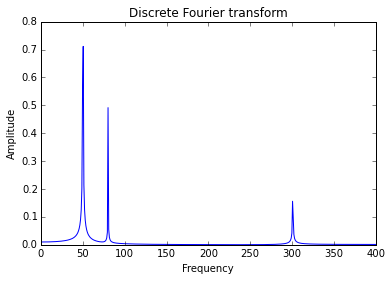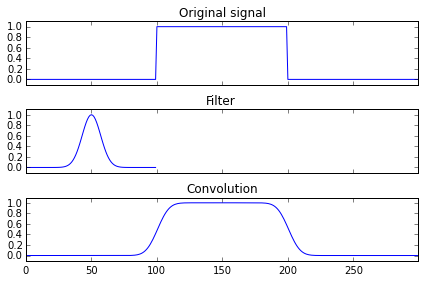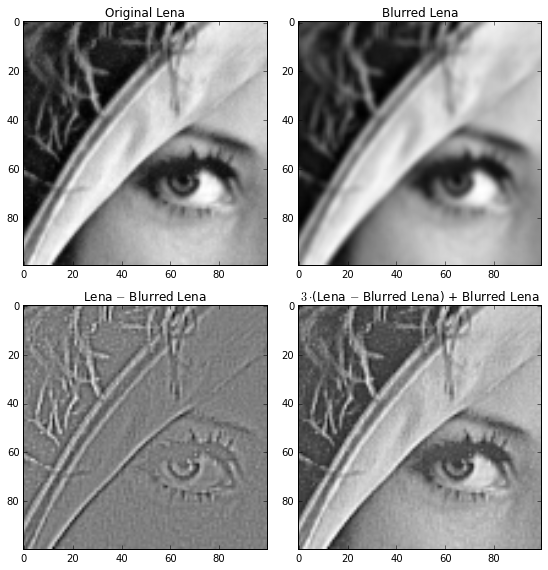# Lecture 4: Matvec complexity, FFT, fast convolution¶

## Matrix-by-vector multiplication (matvec)¶

Multiplication of a matrix $A$ of size $n\times n$ by a vector $x$ of size $n\times 1$: $$y_{i} = \sum_{i=1}^n a_{ij} x_j$$ requires $n^2$ mutliplications and $n(n-1)$ additions. Thus, the overall complexity is $2n^2 - n =$ $\mathcal{O}(n^2)$ .

## How bad is $\mathcal{O}(n^2)$¶

To understand how time-consuming $\mathcal{O}(n^2)$ can be consider the following example. Let $A$ be the matrix of pairwise gravitational interaction between planets in a galaxy. The number of planets in an average galaxy is $10^{11}$, so the size of this matrix will be $10^{11} \times 10^{11}$. To model evolution in time we have to multiply this matrix by vector at each time step. Top supercomputers do around $10^{16}$ floating point operations per second (flops), so the time required to multiply the matrix $A$ by a vector is approximately $$\frac{(10^{11})^2 \text{ operations}}{10^{16} \text{ flops}} = 10^6 \text{ sec} \approx 11.5 \text{ days}$$ for one time step. If we could multiply it with $\mathcal{O}(n)$ complexity, we would get $$\frac{10^{11} \text{ operations}}{10^{16} \text{ flops}} = 10^{-5} \text{ sec}$$ instead. Fortunately, there is an algorithm to be $\mathcal{O}(n)$ for this particular example.

Here is the YouTube video that illustrates collision of two galaxisies which was modelled by $\mathcal{O}(n)$ (or maybe $\mathcal{O}(n \log n)$) algorithm:

In :
from IPython.display import YouTubeVideo

Out:

The question is can we be asymptotically faster for a general matrix $A$?

## Can we beat $\mathcal{O}(n^2)$?¶

Generally speaking no. The point is that we have $\mathcal{O}(n^2)$ input data, so there is no way to be faster for a general matrix. Fortunately, we can be faster for certain types of matrices. Here are some examples:

• The simplest example may be a matrix of all ones, which can be easily multiplied with only $n-1$ additions. This matrix is of rank one. More generally we can multiply fast by low-rank matrices (or by matrices that have low-rank blocks)

• Sparse matrices (contain $\mathcal{O}(n)$ nonzero elements)

• Structured matrices (later this lecture):

• Fourier
• Circulant
• Toeplitz
• Hankel

## Fourier matrix¶

The Fourier matrix is defined as: $$F_n = \begin{pmatrix} 1 & 1 & 1 & \dots & 1 \\ 1 & w^{1\cdot 1}_n & w^{1\cdot 2}_n & \dots & w^{1\cdot (n-1)}_n\\ 1 & w^{2\cdot 1}_n & w^{2\cdot 2}_n & \dots & w^{2\cdot (n-1)}_n\\ \dots & \dots & \dots &\dots &\dots \\ 1 & w^{(n-1)\cdot 1}_n & w^{(n-1)\cdot 2}_n & \dots & w^{(n-1)\cdot (n-1)}_n\\ \end{pmatrix},$$ or equivalently $$F_n = \{ w_n^{kl} \}_{k,l=0}^{n-1},$$ where $$w_n = e^{-\frac{2\pi i}{n}}.$$

Properties:

• Symmetric (not Hermitian!)
• Unitary up to a scaling factor: $F_n^* F_n = F_n F_n^* = nI$ (check this fact). Therefore $F_n^{-1} = \frac{1}{n}F^*_n$
• Can be multiplied by a vector (called dicrete Fourier transform or DFT) with $\mathcal{O}(n \log n)$ complexity (called fast Fourier transform or FFT)! FFT helps to analyze spectrum of a signal and, as we will see later, helps to do fast mutiplications with certain types of matrices.
In :
%matplotlib inline
import matplotlib.pyplot as plt
import numpy as np

N = 1000
dt = 1.0 / 800.0
x = np.linspace(0.0, N*dt, N)
y = np.sin(50.0 * 2.0*np.pi*x) + 0.5*np.sin(80.0 * 2.0*np.pi*x) + 0.2*np.sin(300.0 * 2.0*np.pi*x)
plt.plot(x, y)
plt.xlabel('Time')
plt.ylabel('Signal')
plt.title('Initial signal')

Out:
<matplotlib.text.Text at 0x10dc58450>In :
yf = np.fft.fft(y)
xf = np.linspace(0.0, 1.0/(2.0*dt), N/2)
plt.plot(xf, 2.0/N * np.abs(yf[0:N/2])) #Note: N/2 to N will give negative frequencies
plt.xlabel('Frequency')
plt.ylabel('Amplitude')
plt.title('Discrete Fourier transform')

Out:
<matplotlib.text.Text at 0x10e149350>## Fast Fourier transform (FFT)¶

Here we consider a matrix interpretation of the standard Cooley-Tukey algorithm (1965), which has underlying divide and conquerer idea. Note that in packages more advanced vesions are used.

Let $n$ be a power of 2. First of all we permute the rows of the Fourier matrix such that the first $n/2$ rows of the new matrix had row numbers $1,3,5,\dots,n-1$ and the last $n/2$ rows had row numbers $2,4,6\dots,n$. This permutation can be expressed in terms of multiplication by permutation matrix $P_n$: $$P_n = \begin{pmatrix} 1 & 0 & 0 & 0 & \dots & 0 & 0 \\ 0 & 0 & 1 & 0 &\dots & 0 & 0 \\ \vdots & & & & & & \vdots \\ 0 & 0 & 0 & 0 &\dots & 1 & 0 \\ \hline 0 & 1 & 0 & 0 & \dots & 0 & 0 \\ 0 & 0 & 0 & 1 &\dots & 0 & 0 \\ \vdots & & & & & & \vdots \\ 0 & 0 & 0 & 0 &\dots & 0 & 1 \end{pmatrix},$$

Hence, $$P_n F_n = \begin{pmatrix} 1 & 1 & 1 & \dots & 1 \\ 1 & w^{2\cdot 1}_n & w^{2\cdot 2}_n & \dots & w^{2\cdot (n-1)}_n\\ 1 & w^{4\cdot 1}_n & w^{4\cdot 2}_n & \dots & w^{4\cdot (n-1)}_n\\ \vdots & & & & \vdots\\ 1 & w^{(n-2)\cdot 1}_n & w^{(n-2)\cdot 2}_n & \dots & w^{(n-2)\cdot (n-1)}_n\\ \hline 1 & w^{1\cdot 1}_n & w^{1\cdot 2}_n & \dots & w^{1\cdot (n-1)}_n\\ 1 & w^{3\cdot 1}_n & w^{3\cdot 2}_n & \dots & w^{3\cdot (n-1)}_n\\ \vdots & & & & \vdots\\ 1 & w^{(n-1)\cdot 1}_n & w^{(n-1)\cdot 2}_n & \dots & w^{(n-1)\cdot (n-1)}_n\\ \end{pmatrix},$$ Now let us imagine that we separated its columns and rows by two parts each of size $n/2$.

As a result we get $2\times 2$ block matrix that has the following form $$P_n F_n = \begin{pmatrix} \left\{w^{2kl}_n\right\} & \left\{w_n^{2k\left(\frac{n}{2} + l\right)}\right\} \\ \left\{w_n^{(2k+1)l}\right\} & \left\{w_n^{(2k+1)\left(\frac{n}{2} + l\right)}\right\} \end{pmatrix}, \quad k,l = 0,\dots, \frac{n}{2}-1.$$ So far it does not look like something that works faster :) But we will see that in a minute. Lets have a more precise look at the first block $\left\{w^{2kl}_n\right\}$: $$w^{2kl}_n = e^{-2kl\frac{2\pi i}{n}} = e^{-kl\frac{2\pi i}{n/2}} = w^{kl}_{n/2}.$$ So this block is exactly twice smaller Fourier matrix $F_{n/2}$!

The block $\left\{w_n^{(2k+1)l}\right\}$ can be written as $$w_n^{(2k+1)l} = w_n^{2kl + l} = w_n^{l} w_n^{2kl} = w_n^{l} w_{n/2}^{kl},$$ which can be written as $W_{n/2}F_{n/2}$, where $$W_{n/2} = \text{diag}(1,w_n,w_n^2,\dots,w_n^{n/2-1}).$$

Doing the same tricks for the other blocks we will finally get $$P_n F_n = \begin{pmatrix} F_{n/2} & F_{n/2} \\ F_{n/2}W_{n/2} & -F_{n/2}W_{n/2} \end{pmatrix} = \begin{pmatrix} F_{n/2} & 0 \\ 0 & F_{n/2} \end{pmatrix} \begin{pmatrix} I_{n/2} & I_{n/2} \\ W_{n/2} & -W_{n/2} \end{pmatrix}.$$ Thus, we reduced multiplication by $F_n$ to 2 multiplications by $F_{n/2}$ and cheap multiplications by diagonal matrices. If we apply the obtained expressions recursively to $F_{n/2}$, we will get $\mathcal{O}(n\log n)$ complexity.

In :
#FFT vs full matvec
import time
import numpy as np
import scipy as sp
import scipy.linalg

n = 10000
F = sp.linalg.dft(n)
x = np.random.randn(n)

t = time.time()
y_full = F.dot(x)
print 'Full matvec time =', time.time() - t

t = time.time()
y_fft = np.fft.fft(x)
print 'FFT time =', time.time() - t

print 'Relative error =', (np.linalg.norm(y_full - y_fft)) / np.linalg.norm(y_full)

Full matvec time = 0.171025037766
FFT time = 0.000820159912109
Relative error = 1.53542183088e-12


## Circulant matrices¶

FFT helps to multiply fast by certain types of matrices. We start from a circulant matrix:

$$C = \begin{pmatrix} c_0 & c_{n-1} & c_{n-2} & \dots & c_1 \\ c_{1} & c_{0} & c_{n-1} & \dots & c_2 \\ c_{2} & c_{1} & c_0 & \dots & c_3 \\ \dots & \dots & \dots & \dots & \dots \\ c_{n-1} & c_{n-2} & c_{n-3} & \dots & c_0 \end{pmatrix}$$

Theorem. Let $C$ be a circulant matrix of size $n\times n$ and let $c$ be it's first column , then $$C = \frac{1}{n} F_n^* \text{diag}(F_n c) F_n$$

Proof. Consider a number $$\lambda (\omega) = c_0 + \omega c_1 + \dots + \omega^{n-1} c_{n-1},$$ where $\omega$ is any number such that $\omega^n=1$. Lets multiply $\lambda$ by $1,\omega,\dots, \omega^{n-1}$: $$\begin{split} \lambda & = c_0 &+& \omega c_1 &+& \dots &+& \omega^{n-1} c_{n-1},\\ \lambda\omega & = c_{n-1} &+& \omega c_0 &+& \dots &+& \omega^{n-1} c_{n-2},\\ \lambda\omega^2 & = c_{n-2} &+& \omega c_{n-1} &+& \dots &+& \omega^{n-1} c_{n-3},\\ &\dots\\ \lambda\omega^{n-1} & = c_{1} &+& \omega c_{2} &+& \dots &+& \omega^{n-1} c_{0}. \end{split}$$ Therefore, $$\lambda(\omega) \cdot \begin{pmatrix} 1&\omega & \dots& \omega^{n-1} \end{pmatrix} = \begin{pmatrix} 1&\omega&\dots& \omega^{n-1} \end{pmatrix} \cdot C.$$ Writing this for $\omega = 1,w_n, \dots, w_n^{n-1}$ we get $$\Lambda F_n = F_n C$$ and finally $$C = \frac{1}{n} F^*_n \Lambda F_n, \quad \text{where}\quad \Lambda = \text{diag}(F_nc) \qquad\blacksquare$$

#### Fast matvec with circulant matrix¶

Representation $C = \frac{1}{n} F^* \text{diag}(F_n c) F_n$ gives us an explicit way to multiply a vector $x$ by $C$ in $\mathcal{O}(n\log n)$ operations. Indeed, $$Cx = \frac{1}{n} F_n^* \text{diag}(F_n c) F_n x = \text{ifft}\left( \text{fft}(c) \circ \text{fft}(x)\right)$$ where $\circ$ denotes elementwise product (Hadamard product) of two vectors (since $\text{diag}(a)b = a\circ b$) and ifft denotes inverse Fourier transform $F^{-1}_n$.

In :
import time
import numpy as np
import scipy as sp
import scipy.linalg

def circulant_matvec(c, x):
return np.fft.ifft(np.fft.fft(c) * np.fft.fft(x))

n = 10000
c = np.random.random(n)
C = sp.linalg.circulant(c)
x = np.random.randn(n)

t = time.time()
y_full = C.dot(x)
print 'Full matvec time =', time.time() - t

t = time.time()
y_fft = circulant_matvec(c, x)
print 'FFT time =', time.time() - t

print 'Relative error =', (np.linalg.norm(y_full - y_fft)) / np.linalg.norm(y_full)

Full matvec time = 0.0920350551605
FFT time = 0.00724005699158
Relative error = 5.74383700118e-15


## Toeplitz matrices¶

Another example where FFT can help us with the fast matvec operation is a Toeplitz matrix (diagonal constant matrix): $$T = \begin{pmatrix} t_0 & t_{-1} & t_{-2} & t_{-3}& \dots & t_{1-n} \\ t_{1} & t_{0} & t_{-1} & t_{-2}& \dots & t_{2-n} \\ t_{2} & t_{1} & t_0 & t_{-1} &\dots & t_{3-n} \\ t_{3} & t_{2} & t_1 & t_0 & \dots & t_{4-n} \\ \dots & \dots & \dots & \dots & \dots & \dots\\ t_{n-1} & t_{n-2} & t_{n-3} & t_{n-4} &\dots &t_0 \end{pmatrix},$$ or equivalently $T_{ij} = t_{i-j}$.

Matvec operation can be written as $$y_i = \sum_{j=1}^n t_{i-j} x_j,$$ which can be interpreted as a discrete convolution of filter $t_i$ and signal $x_i$. For simplicity the size of the filter $t$ is such that the sizes of the input and output signals are the same. Generally, filter size can be arbitrary.

Fast convolution computation has a variety of applications, for instance, in signal processing or partial differential and integral equations. For instance, here is the smoothing of a signal:

In :
from scipy import signal
alpha = 0.01
sig = np.repeat([0., 1., 0.], 100)
filt = np.exp(-alpha * (np.arange(100)-50)**2)
filtered = signal.convolve(sig, filt, mode='same') / sum(filt)

fig, (ax_orig, ax_filt, ax_filtered) = plt.subplots(3, 1, sharex=True)
ax_orig.plot(sig)
ax_orig.margins(0, 0.1)
ax_filt.plot(filt)
ax_filt.margins(0, 0.1)
ax_filtered.plot(filtered)
ax_filtered.margins(0, 0.1)

ax_orig.set_title('Original signal')
ax_filt.set_title('Filter')
ax_filtered.set_title('Convolution')

fig.tight_layout()#### Fast matvec with Toeplitz matrix¶

The multiplication by a Toeplitz matrix can be reduced to the multiplication by a circulant. Indeed, every Toeplitz matrix of size $n\times n$ can be embedded into a Circulant matrix $C$ of size $2n-1 \times 2n-1$: $$C = \begin{pmatrix} T & \dots \\ \dots & \dots \end{pmatrix}.$$ The $3\times 3$ matrix $T = \begin{pmatrix} t_0 & t_{-1} & t_{-2} \\ t_{1} & t_{0} & t_{-1} \\ t_{2} & t_{1} & t_0 \\ \end{pmatrix}$ can be embedded as follows $$C = \begin{pmatrix} t_0 & t_{-1} & t_{-2} & t_{2} & t_{1}\\ t_{1} & t_{0} & t_{-1} & t_{-2} & t_{2} \\ t_{2} & t_{1} & t_0 & t_{-1} & t_{-2} \\ t_{-2}& t_{2} & t_{1} & t_0 & t_{-1} \\ t_{-1} & t_{-2} & t_{2} & t_{1} & t_0 \end{pmatrix}.$$ For matvec $\begin{pmatrix} y_1 \\ y_2 \\ y_3 \end{pmatrix}= \begin{pmatrix} t_0 & t_{-1} & t_{-2} \\ t_{1} & t_{0} & t_{-1} \\ t_{2} & t_{1} & t_0 \\ \end{pmatrix} \begin{pmatrix} x_1 \\ x_2 \\ x_3 \end{pmatrix}$ we pad vector $x$ with zeros: $$\begin{pmatrix} y_1 \\ y_2 \\ y_3 \\ \star \\ \star \end{pmatrix} = \begin{pmatrix} t_0 & t_{-1} & t_{-2} & t_{2} & t_{1}\\ t_{1} & t_{0} & t_{-1} & t_{-2} & t_{2} \\ t_{2} & t_{1} & t_0 & t_{-1} & t_{-2} \\ t_{-2}& t_{2} & t_{1} & t_0 & t_{-1} \\ t_{-1} & t_{-2} & t_{2} & t_{1} & t_0 \end{pmatrix} \begin{pmatrix} x_1 \\ x_2 \\ x_3 \\ 0 \\ 0 \end{pmatrix}= \text{ifft}(\text{fft}(\begin{pmatrix} t_0 \\ t_{1} \\ t_{2} \\ t_{-2} \\ t_{-1} \end{pmatrix})\circ \text{fft}(\begin{pmatrix} x_1 \\ x_2 \\ x_3 \\ 0 \\ 0 \end{pmatrix})).$$ Note that you do not need to form and store the whole matrix $T$.

## Multilevel Toeplitz matrices¶

The 2-dimensional convolution is defined as $$y_{i_1i_2} = \sum_{j_1,j_2=1}^n t_{i_1-j_1, i_2-j_2} x_{j_1 j_2}.$$ Note that $x$ and $y$ are 2-dimensional arrays and $T$ is 4-dimensional. To reduce this expression to matrix-by-vector product we have to reshape $x$ and $y$ into long vectors: $$\text{vec}(x) = \begin{pmatrix} x_{11} \\ \vdots \\ x_{1n} \\ \hline \\ \vdots \\ \hline \\ x_{n1} \\ \vdots \\ x_{nn} \end{pmatrix}, \quad \text{vec}(y) = \begin{pmatrix} y_{11} \\ \vdots \\ y_{1n} \\ \hline \\ \vdots \\ \hline \\ y_{n1} \\ \vdots \\ y_{nn} \end{pmatrix}.$$ In this case matrix $T$ is block Toeplitz with Toeplitz blocks: $$T = \begin{pmatrix} T_0 & T_{-1} & T_{-2} & \dots & T_{1-n} \\ T_{1} & T_{0} & T_{-1} & \dots & T_{2-n} \\ T_{2} & T_{1} & T_0 & \dots & T_{3-n} \\ \dots & \dots & \dots & \dots & \dots\\ T_{n-1} & T_{n-2} & T_{n-3} &\dots &T_0 \end{pmatrix}, \quad \text{where} \quad T_k = t_{k, i_2 - j_2}\quad \text{are Toeplitz matrices}$$

#### Fast matvec with multilevel Toeplitz matrix¶

To get fast matvec we need to embed block Toeplitz matrix with Toeplitz blocks into the block circulant matrix with circulant blocks. The analog of $$\begin{pmatrix} y_1 \\ y_2 \\ y_3 \\ \star \\ \star \end{pmatrix} = \text{ifft}(\text{fft}(\begin{pmatrix} t_0 \\ t_{1} \\ t_{2} \\ t_{-2} \\ t_{-1} \end{pmatrix})\circ\text{fft}(\begin{pmatrix} x_1 \\ x_2 \\ x_3 \\ 0 \\ 0 \end{pmatrix})).$$ will look like $$\begin{pmatrix} y_{11} & y_{12} & y_{13} & \star & \star \\ y_{21} & y_{22} & y_{23} & \star & \star \\ y_{31} & y_{32} & y_{33} & \star & \star \\ \star & \star & \star & \star & \star \\ \star & \star & \star & \star & \star \\ \end{pmatrix} = \text{ifft2d}(\text{fft2d}(\begin{pmatrix} t_{0,0} & t_{1,0} & t_{2,0} & t_{-2,0} & t_{-1,0} \\ t_{0,1} & t_{1,1} & t_{2,1} & t_{-2,1} & t_{-1,1} \\ t_{0,2} & t_{1,2} & t_{2,2} & t_{-2,2} & t_{-1,2} \\ t_{0,-2} & t_{1,-2} & t_{2,-2} & t_{-2,-2} & t_{-1,-2} \\ t_{0,-1} & t_{1,-1} & t_{2,-1} & t_{-2,-1} & t_{-1,-1} \end{pmatrix}) \circ \text{fft2d}(\begin{pmatrix}x_{11} & x_{12} & x_{13} & 0 & 0 \\ x_{21} & x_{22} & x_{23} & 0 & 0 \\ x_{31} & x_{32} & x_{33} & 0 & 0 \\ 0 & 0 & 0 & 0 & 0 \\ 0 & 0 & 0 & 0 & 0 \\ \end{pmatrix})),$$ where fft2d is 2-dimensional fft that consists of one-dimensional transforms, applied first to rows and and then to columns (or vice versa).

In :
# Blurring and Sharpening the Lena by convolution

from scipy import signal
from scipy import misc

filter_size = 3
filter_blur = np.ones((filter_size, filter_size)) / filter_size**2
lena = misc.lena()

blurred = signal.convolve2d(lena, filter_blur, boundary='symm', mode='same')

fig, ax = plt.subplots(2, 2, figsize=(8, 8))
ax[0, 0].imshow(lena[200:300, 200:300], cmap='gray')
ax[0, 0].set_title('Original Lena')
ax[0, 1].imshow(blurred[200:300, 200:300], cmap='gray')
ax[0, 1].set_title('Blurred Lena')
ax[1, 0].imshow((lena - blurred)[200:300, 200:300], cmap='gray')
ax[1, 0].set_title('Lena $-$ Blurred Lena')
ax[1, 1].imshow(((lena - blurred)*3 + blurred)[200:300, 200:300], cmap='gray')
ax[1, 1].set_title('$3\cdot$(Lena $-$ Blurred Lena) + Blurred Lena')
fig.tight_layout()## Conclusions¶

• For big matrices $\mathcal{O}(n^2)$ is very time-consuming
• Can be better for special types of matrices